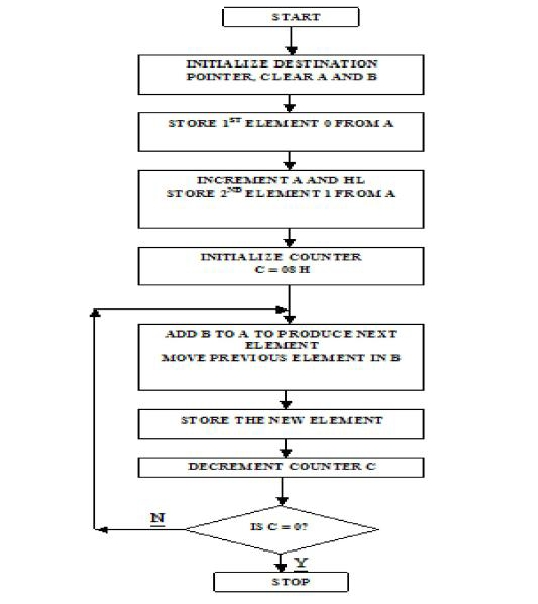# 8085 program to generate Fibonacci sequence

In this program we will see how to generate Fibonacci sequence.

### Problem Statement

Write 8085 Assembly language program to generate the first ten elements of the Fibonacci sequence using registers only and store them in memory locations 8050H to 8059H

### Discussion

This program will generate the Fibonacci numbers. The Fibonacci numbers follows this relation F(i) = F(i - 1) + F(i - 2) for all i >2 with F(1) = 0, F(2) = 1.

### Input

In this case we are not providing any input, this program will generate ten Fibonacci numbers.

### Flow Diagram### Program

800021, 50, 80STARTLXI H 8050H Pointer to the OUT-BUFFER
8003AF
XRA A Clear accumulator and reg. B
800447
MOV B, A
800577
MOV M, A Copying content to the target location
80063C
INR A Increment A
800723
INX H Go to the next dest. address.
800877
MOV M, A Moving the content
80 09 0E, 08
MVI C, 08H Initialize counter
800B 80LOOPADD B Getting the next term
800C 46
MOV B, M Initializing term e.g. F1 = F2
800D 23
INX H Go to the next dest. address.
800E 77
MOV M, A Writing to the OUT-BUFFER
800F 0D
DCR C Decrement count until 0 is reached F3= F1 + F2 (A) = (A) + (B) This is done with instruction ADDB.
8010C2, 0B, 80
JNZ LOOP
801376
HLT Terminate the program

......
805000
805101
805201
805302
805403
805505
805608
80570D
805815
805922
......